MP Board Class 7th Maths Solutions Chapter 15 Visualising Solid Shapes Ex 15.1

Question 1.
Identify the nets which can be used to make cubes (cut out copies of the nets and try it):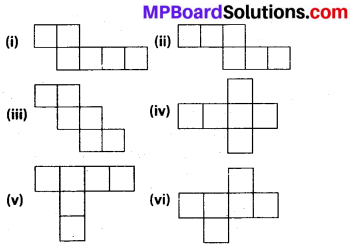Solution:
(i) The given net can be folded as follows: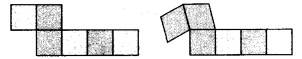When the faces are folded to make a cube, they will be overlapping each other.

(ii) The given net can be folded as follows: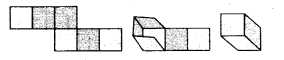A cube can thus be formed in the above way.

(iii) The given net can be folded as follows: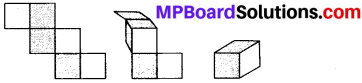A cube can thus be formed in the above way.

(iv) The given net can be folded as follows: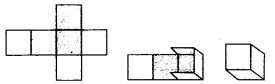A cube can thus be formed in the above way.

(v) The given net can be folded as follows: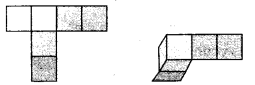When the faces are folded to make a cube, they will be overlapping each other.

(vi) The given net can be folded as follows: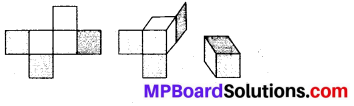A cube can thus be formed in the above way.

Question 2.
Dice are cubes with dots on each face. Opposite faces of a die always have a total of seven dots on them.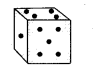Here are two nets to make dice (cubes); the numbers inserted in each square indicate the number of dots in that box.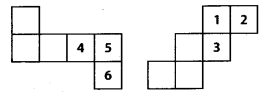Insert suitable numbers in the blanks, remembering that the number on the opposite faces should total to 7.
Solution:
(i) The numbers can be inserted as follows so as to make the given net into a net of a dice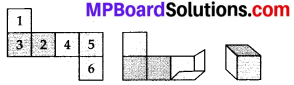It can be observed that the sum of the opposite faces is 7.

(ii) The numbers can be inserted as follows so as to make the given net into a net of a dice.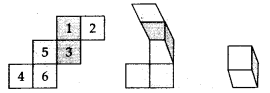It can be observed that the sum of the opposite faces is 7.Question 3.
Can this be a net for a die?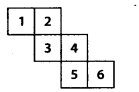Solution:
The given net can be folded as follows.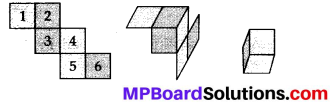It can be observed that the opposite faces of the die so formed have 2 and 5, 1 and 4, 3 and 6 on them. The sum of the numbers on the opposite faces comes to 7, 5 and 9 respectively. However, in case of a die, the sum of the numbers on the opposite faces should be 7. Hence, this net is not for a die.

Question 4.
Here is an incomplete net for making a cube. Complete it in at least two different ways. Remember that a cube has six faces. How many are there in the net here? (Give two separate diagrams. If you like, you may use a squared sheet for easy manipulation.)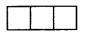Solution:
There are 3 faces in the given net.
The given net can be completed as follows.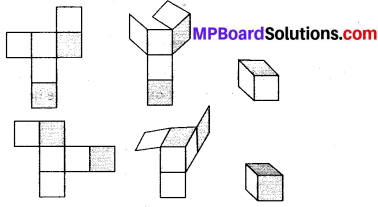Question 5.
Match the nets with appropriate solids: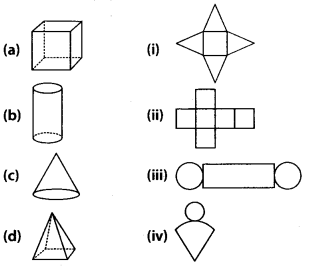Solution:
(a) It can be unfolded as follows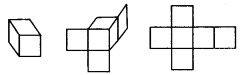Hence, (ii) is a correct matching option.

(b) It can be unfolded as follows: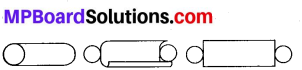Hence, (iii) is the correct matching option,

(c) It can be unfolded as follows: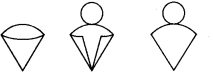Hence, (iv) is the correct matching option,

(d) It can be unfolded as follows: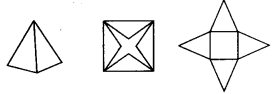Hence, (i) is the correct matching option.
Therefore, (a) – (ii), (b) – (iii), (c) – (iv), (d) – (i)